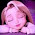Tuesday, 21 January 2014

MULTILEVEL HIERARCHY

Creating multilevel hierarchy in java

In simple inheritance a subclass or derived class derives the properties from its parent class, but in multilevel inheritance a subclass is derived from a derived class. One class inherits only single class. Therefore, in multilevel inheritance, every time ladder increases by one. The lower most class will have the properties of all the super classes’.

It is common that a class is derived from another derived class.The class student serves as a base class for the derived class marks, which in turn serves as a base class for the derived class percentage.The class marks is known as intermediates base class since it provides a link for the inheritance between student and percentage.The chain is known as inheritance path. When this type of situation occurs, each subclass inherits all of the features found in all of its super classes. In this case, percentage inherits all aspects of marks and student.

Example for multilevel hierarchy:

class student
{
int rollno;
String name;

student(int r, String n)
{
rollno = r;
name = n;
}
void dispdatas()
{
System.out.println("Rollno = " + rollno);
System.out.println("Name = " + name);
}
}

class marks extends student
{
int total;
marks(int r, String n, int t)
{
super(r,n);   //call super class (student) constructor
total = t;
}
void dispdatam()
{
dispdatas();    // call dispdatap of student class
System.out.println("Total = " + total);
}
}

class percentage extends marks
{
int per;

percentage(int r, String n, int t, int p)
{
super(r,n,t);  //call super class(marks) constructor
per = p;
}
void dispdatap()
{
dispdatam();    // call dispdatap of marks class
System.out.println("Percentage = " + per);
}
}
class Multi_Inhe
{
public static void main(String args[])
{
percentage stu = new percentage(102689, "RATHEESH", 350, 70); //call constructor percentage
stu.dispdatap();  // call dispdatap of percentage class
}
}

Output:
Rollno = 102689
Name = RATHEESH
Total = 350
Percentage = 70

1.2.3.